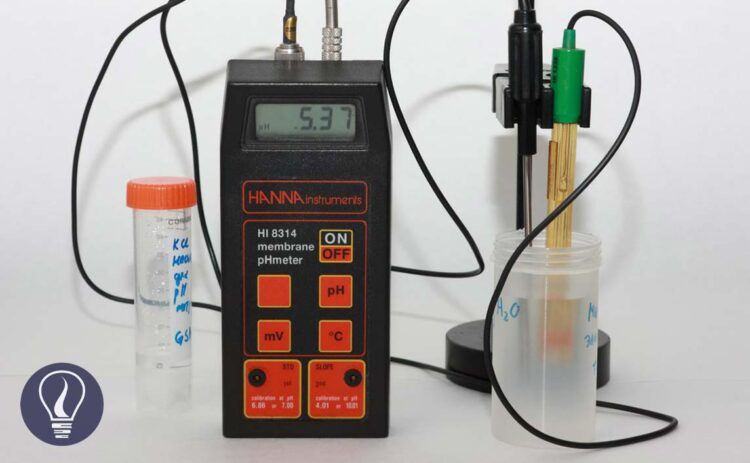More results...

Generic selectors
Exact matches only
Search in title
Search in content
Post Type Selectors

# How to Measure pH in WaterB.Sc (Hons, USJ) (Polymer Science and Technology, Chemistry, Physics)
Categories
Last Updated On : February 22, 2023
Published Date : January 23, 2023

## How to Measure pH in Water sample?

pH value is a measurement of H+ ions in a medium. It tells us how acidic or basic the medium is. Generally, the following equation is used to calculate the pH value.

But when we are measuring pH using a pH meter, we are attempting to calculate the negative logarithm of the hydrogen ion activity, not its concentration. Therefore, it is better to express the pH value as follows.

Where,

• [H+] – Concentration of H+ ions
• γH+ - activity coefficient

Measuring pH is the determination of the activity of H+ ions in the solution. A standard hydrogen electrode and reference electrode are used for the measurement. The hydrogen electrode contains a platinum electrode and Hydrogen gas bubbled across the platinum electrode at 1 atm of pressure. But in pH meters, a glass electrode is used due to the difficulty of using hydrogen electrodes. As a reference electrode in the pH meter, a silver (Ag)-silver (AgCl) chloride electrode is used.

There is a linear relationship between the electromotive force (emf) produced in the glass electrode system and pH. We can plot emf vs pH charts for known pH buffers. Sample pH is determined by the extrapolation of the chart.

Before measuring the pH of the sample, the pH measuring instrument should be calibrated. We use National Institute of Standards and Technology (NIST) buffers to calibrate the instrument.

Where:

• pHB - assigned pH of NIST buffer.
• aH+ - hydrogen ion activity.

Using the above operation, we can find the pH of the sample as follows.

where:

• pHx - potentiometrically measured sample pH,
• F - Faraday: 9.649*104 coulomb/mole,
• Ex - sample emf, V,
• Es - buffer emf, V,
• R - gas constant; 8.314 joule/ (mole K), and
• T - absolute temperature, K.

## Experimental method of measuring pH in water samples

To measure pH in water samples, pH meters are used. pH meter is an instrument that contains a potentiometer, a glass electrode, a reference electrode, and a temperature-compensating device. Generally, we can measure the pH by immersing this circuit in the test solution.

### Preparation of standard buffers

Before measuring the pH of the sample, the first thing to do is calibrate the pH meter. To calibrate, standard buffers are used.

Above chemicals are dissolved in distilled water at 25°C and diluted to 1000 ml.

### Calibration of pH meter

Electrodes of the pH meter are stored in a specific solution. The type of solution may be varied according to the type of electrode. But generally, electrodes are stored in a solution that has a conductivity greater than 4000 μmhos/cm. the best storage solution for single glass electrodes is a pH 4 buffer.

A saturated KCl solution is best for storing calomel and Ag/AgCl reference electrodes. When the pH meter is taken for calibration, the first electrodes are removed from the storage solution. Then the electrodes are rinsed with distilled water and blot dry with soft tissues.

The dried electrodes are placed in the first buffer solution and set at the iso-electric point. The iso-electric point is the pH value where the temperature has no effect on the potential of the electrode. The electrodes are removed from the buffer, rinsed, and blot dried.

Then they are placed in a second buffer solution. The buffer solutions should be at a fixed temperature which may be room temperature. Such as 25°C. Or the temperature may be the sample temperature.

### Measure pH in a water sample

The calibrated electrodes are immersed in the fresh sample for measuring pH. To ensure the homogeneity of the sample and establish the equilibrium between the sample and the electrodes, the sample is stirred constantly.

But the stirring should be done gently to minimize carbon dioxide entrainment. The reading is taken as the pH of the sample.## References and Attributes

National Institute of Standards and Technology (NIST) - Standardization of pH Measurements

### Figures:

The cover image was designed using an image by Sergei Golyshev from Russia, licensed under CC BY-SA 2.0, via Wikimedia Commons